If you have been following the first two posts (post 1, post 2), now is time to reap the rewards! I will show here how to obtain a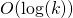-competitive algorithm for (weighted) paging, i.e., when the metric space corresponds to the leafs of a weighted star. This was viewed as a breakthrough result 10 years ago (with a JACM publication by Bansal, Buchbinder and Naor in 2012), and for good reasons as this simplest instance of-server was in fact the one studied in the seminal paper by Sleator and Tarjan in 1985 which introduced the competitive analysis of online algorithms (actually to be precise Sleator and Tarjan considered the unweighted case, for which aalgorithm was known much before).

State space for weighted-paging

Letbe the weight of the edge from leafto the root. Recall from the previous post that we want to find a norm and a convex body that can express the Wasserstein-distance between two fractional-server configurations.

Consider a fractional move fromto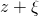. Then clearly, the amount of masshas to transfer through the edge, so that the Wasserstein-distance is at least. Furthermore there is trivially a transport plan achieving that much total mass transfer. In other words we just proved that in this case the appropriate norm is a weighted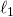norm (namely) and one can simply use the basic state space(recall from the previous post that we have to work with anticonfiguration, and that the mapping to a configuration is simply given by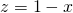).

Applying the general mirror descent framework

Given a request at location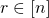and a current anticonfiguration, our proposed (fractional) algorithm is to run the mirror descent dynamics with a continuous time linear costfrom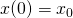(i.e.,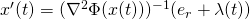for some) and until the first timeat which(i.e., one has a server at location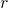in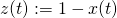). By the lemma at the end of the previous post one has (denote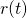for the request being serviced at time)One can think of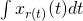as a “virtual service cost”. In-server this quantity has no real meaning, but the above inequality shows that this quantity, which only depends on the algorithm, is tightly related to the value of OPT (provided thathas a small Lipschitz norm). Thus we see that we have two key desiderata for the choice of the mirror map(i) it should have a small Lipschitz norm, (ii) one should be able to relate the movement costto this “virtual service cost”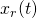, say up to a multiplicative factor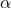. One would then obtain a-competitive algorithm.

Entropy regularization for weighted-paging

Let us look at (ii), and we shall see that the entropy regularization comes out very naturally. Ignore for a moment the Lagrange multiplierand let us search for a separable regularizer of the form. We want to relateto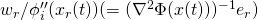. Making those two quantities equal givesand thus the regularizer is a weighted negentropy: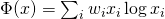.

We now need to verify that this relation between the virtual service cost and the movement cost remains true even when the Lagrange mutilplieris taken into account. Note that because of the form of the state spacethe multiplier contains a term of the form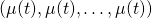(which corresponds to the constraint) and for each location a term forcing the derivative to beif the value of the missing mass has reached. In other words we obtain the following dynamics for mirror descent with the weighted negentropy regularization:Notice that up to a factor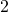one can focus on controlling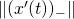(that is only movement into a location is charged). In that view the Lagrange multipliers simply have no effect, since one has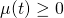(indeed recall that). Thus we see that the movement costis exactly bounded by the virtual service costin this case.

MakingLipschitz

It remains to deal with a non-trivial issue, namely the entropy is not Lipschitz on the simplex! A similar issue is faced in online learning when one tries to prove tracking expert regret bounds, i.e., bounds with respect to a shifting expert. The standard solution (perhaps first used by Herbster and Warmuth in 98, see also Blum and Burch 00) is to shift the variables so that they never get below some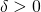, in which case the Lipschitz constant would be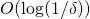. In the-server scenario one can stop the dynamics when(instead of) provided that the mapping fromto the actual fractional configuration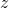is now given by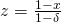. This raises a final issue: the total amount of server mass in such ais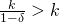. Next we show that if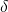is small enough thencan be “rounded” online to a fractional-server configuration at the expense of a multiplicativemovement. Precisely we show that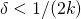is sufficient, which in turns gives a final competitive ratio offor weighted-paging.

Fromservers toservers

Consider a fractional server configurationwith total mass(i.e.,), which we want to transform into a server configuration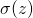with total mass. A natural way to “round down” is at each location to putif the mass was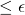. Furthermore a mass ofshould stay. This suggests the mapping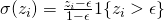, which is-Lipschitz. Thus the movement ofis controlled by the movement ofup to a multiplicative factor. Moreover one clearly has(in fact the inequality can be strict, in which case one should think of the “lost mass” as being stored at the root, this incurs no additional movement cost).